PSAT Math : Sectors

Example Questions

1 3 Next →

Example Question #3 : How To Find The Angle Of A Sector

What is the angle of a sector that has an arc length of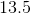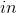on a circle of diameter?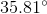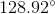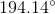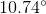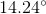Explanation:

The first thing to do for this problem is to compute the total circumference of the circle. Notice that you were given the diameter. The proper equation is therefore: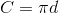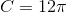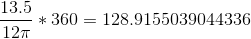Rounded to the nearest hundredth, this is.